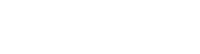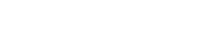SkyCiv Documentation

Your guide to SkyCiv software - tutorials, how-to guides and technical articles

1. Home
2. SkyCiv Retaining Wall
3. Articles and Tutorials
4. Coefficient of Friction for Retaining Wall Design

# Coefficient of Friction for Retaining Wall Design

## What is Friction

Friction is a resisting force that is generated when two bodies interact, typically one sliding across the other. You might remember from your school physics lessons that there are two classes of friction, static and kinetic. Static friction is the frictional force generated to prevent any movement between the two bodies whilst kinetic friction is the frictional force generated when the two bodies are in motion.

For retaining wall design, we consider the wall to be stationary, therefore we are dealing with static friction. Moreover, to ensure the wall meets the required factor of safety, we must consider the frictional force that is generated between the ground and the underside of the Retaining Wall footing in our calculations. The frictional force under the footing assists our design to resist the sliding failure mode of a Retaining Wall.

## How to Calculate Frictional Force

The equation to calculate frictional force is very straightforward. The trickier part might be trying to determine an appropriate friction coefficient.Where:

$${F}_{f}$$ = Frictional Force

$$μ$$ = Coefficient of friction

$${W}_{t}$$ = Total weight contributing to frictional force

Let’s explore the logic of this equation. Consider a box sitting on the floor with a weight of 1kN. We know that the frictional force is the product of μ and the total contributing weight. Just for a moment, let’s give μ a value of 1 essentially removing it from the equation. This means that the frictional force is now equal to the total weight. If the total weight of our box was 1 kN then so is the frictional force. If we try to slide that box across the floor, we would need to apply >1kN to the side of the box for it to overcome the static frictional resistance and start to slide.

Say our box is made of cardboard and the floor is constructed with timber floorboards. Now we may recognize that in reality, we won’t need to push that hard to move the box. This is because the coefficient of friction between our box and the floor is more likely to be significantly less than 1. As we lower the coefficient of friction, we can then apply less force to the side of the box to overcome frictional resistance.

Our final definition of the coefficient of friction might be something like this: The coefficient of friction is the value that defines how much of the total contributing weight translates to the frictional force which prevents a body from sliding across another surface.

## Considering Friction in Design

If we consider one of the most common types of retaining walls, a Cantilever Retaining Wall, we must consider three major forces that will interact with one another to balance the sliding forces. These include passive earth pressure, frictional resistance, and active earth pressure.In the diagram above, we must ensure that [Passive Forces + Frictional Force > Active Forces] to ensure sliding does not occur. We can calculate the total weight as the weight of the soil and water above the footing and, the self-weight of the wall. Then we must choose an appropriate coefficient of friction, to final the final frictional force.

In theory, μ could be any value from 0 to 1 however, in design, it is typical for the coefficient of friction to be taken as 0.5. This might be increased up to 2/3 which (in most cases) will cause the calculation to be less conservative. The most accurate value might be obtained by running some quick tests of the soil on site.

Another commonly accepted way to determine the coefficient of friction is to substitute a soil’s internal angle of friction into the following equation:Where:

μ = Coefficient of friction

φ = Internal angle of friction

Some common values for the internal angle of friction of various soils include 30-40 (sand), 35 (gravel), 34 (silt) and, 20 (clay). Plotting these values we obtain the following chart.## Summary

We revisited our definition of friction to recognize that we typically deal with static friction in retaining wall design. Following that, we then had a look at the equation to calculate frictional force. Considering cases of higher and lower coefficients of friction we were able to discuss discussed what kind of effects this would have on trying to push an object across the floor.

We then considered how this phenomenon occurs in Retaining Walls and what it means for us as designers. We then wrapped up by looking at commonly accepted values to use for the coefficient of friction in Retaining Wall design and an easy equation that allows us to approximate the coefficient of friction given the internal angle of friction for a soil.

You can try out SkyCiv’s Retaining Wall Calculator, it’s free to use! Under the paid version, users can change the Coefficient of Friction to see how it affects your designs using our detailed design calculation reports.Steve Richardson
API Manager - Australia/NZ
BEng (Civil)